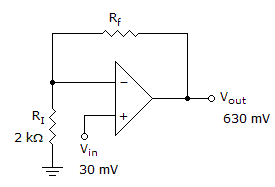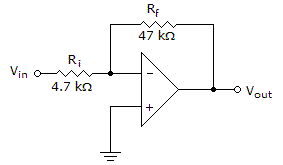# Electronics - Operational Amplifiers

### Exercise :: Operational Amplifiers - Filling the Blanks

6.

An op-amp circuit in which the output voltage is equal to the difference between the two input voltages is called a(n) _____

 A. integrator B. differentiator C. differential amplifier D. voltage regulator

Explanation:

No answer description available for this question. Let us discuss.

7.The feedback resistor has a value of ______ in the given circuit

 A. 2 kB. 10.5 kC. 21 kD. 40 kExplanation:

No answer description available for this question. Let us discuss.

8.The input impedance is ______ for the amplifier in the given circuit. The data sheet values are Zin = 3 M, Zout = 80, and Aol = 250,000.

 A. 4.7 kB. 22.7 kC. 3 MD. 68.6 kExplanation:

No answer description available for this question. Let us discuss.

9.

The Q of a crystal _________.

 A. is extremely low B. is about 40 or so in most cases C. is extremely high D. none of the above

Explanation:

No answer description available for this question. Let us discuss.

10.

The 555 timer can be connected to operate a(n) ___________.

 A. astable multivibrator B. bistable multivibrator C. monostable multivibrator D. astable multivibrator and a monostable multivibrator